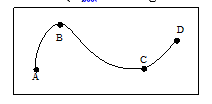Assertion (A) : In the following fig. The distance travelled by the particle is the length of the            curve ABCD            Reason (R) : The length of the path covered by a particle is called distance

# Assertion (A) : In the following fig. The distance travelled by the particle is the length of the            curve ABCD            Reason (R) : The length of the path covered by a particle is called distance1. A

Both A and R are true and R is the correct explanation of the A

2. B

Both A and R are true but R is not the correct explanation of the A.

3. C

A is correct, but R is incorrect

4. D

A is incorrect, but R is correct

Register to Get Free Mock Test and Study Material

+91

Verify OTP Code (required)

### Solution:

The distance travelled by the particle is the length of the curve ABCD.

The length of the path covered by a particle is called distance.Register to Get Free Mock Test and Study Material

+91

Verify OTP Code (required)